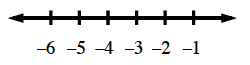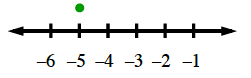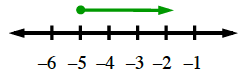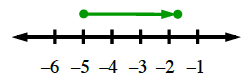### Home > CCAA > Chapter 2 Unit 2 > Lesson CC2: 2.2.2 > Problem2-53

2-53.

Use a number line to show your work as you find the value of each expression.

1. $-5+3.25$

1. $3.18+(−7)$

1. $-5+3.25$

Draw a number line. A number line, with equally placed marks, labeled from left to right as follows: negative 6, negative 5, negative 4, negative 3, negative 2, negative 1.

Draw a point above the first number in the expression.Added: a closed circle above negative 5.

Is the number being added positive? If so, draw an arrow going in the positive direction. If the second number is negative, draw the arrow going in the negative direction. The arrow's length is the second number. Added: An arrow starting at the closed circle above negative 5, and pointing right, going just past negative 2.

The point where the line ends is the answer. Added: A closed circle above and to the right of negative 2.$-1.75$

2. $3.18+(−7)$

Follow the steps given in the help for part (a).